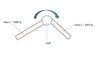# Torque for rotating a shaft of unbalanced weight

• sekar_507

#### sekar_507

1. Hi all. Can anyone please help me find the torque required for rotating a shaft which has two masses 1000 Kg each at 120 Degree angles. Please refer to the attachment for clarity.

## The Attempt at a Solution

#### Attachments

•Torque calc.JPG
11.6 KB · Views: 770
I think you need the lengths of the two bars.

The bars are 500mm long each. How do I calculate the torque?

Torque = force times length.
So, what do you think you should use for length and force?

You sure the bars are of equal length? Looks like bar 1 is longer than bar 2.

Last edited:
should i use formula torque = I * Angular velocity for calculating the torque for rotating the shaft? Please clarify

No, since that's the formula for angular momentum, not torque.

ok then. since both the masses are at 120 deg angle. what is the total mass i should i consider. should i add 1000+1000 = 2000 Kg or should i calculate the resultant mass? can u help me in finding the resultant mass?

You never answered my question: are you sure the masses are of equal length?

Yes. Both the masses are of equal length.

OK, (though I'm still wondering why the drawing shows them as clearly of different lengths).

How about writing an expression for the torque about the axis of rotation as a function of angular position? You can call zero angle at any position you like.

Take one bar at a time. What's the torque gravity applies to one of the bars as a function of its angular position?

Last edited: